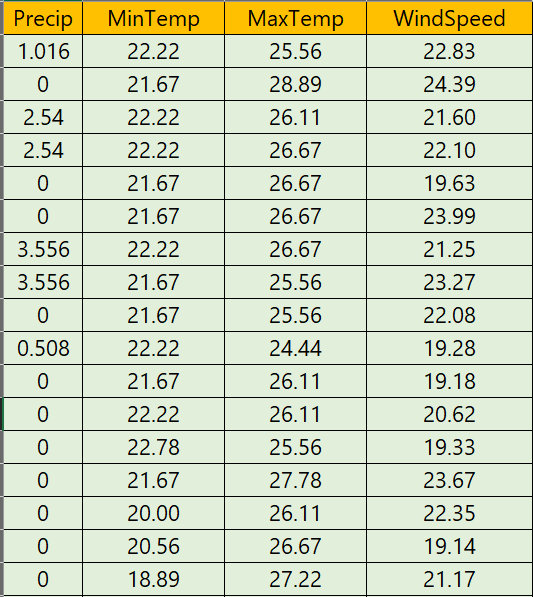# Linear Regression in Python: Sklearn vs ExcelIs Microsoft excel a good alternative for a quick and approximate linear regression prediction business case? I think yes, but let’s do a…Around 13 years ago, Scikit-learn development started as a part of Google Summer of Code project by David Cournapeau. As time passed Scikit-learn became one of the most famous machine learning library in Python. It offers several classifications, regression and clustering algorithms and its key strength, in my opinion, is seamless integration with Numpy, Pandas and Scipy.

Around 13 years ago, Scikit-learn **development started as a part of **Google Summer of Code project by David Cournapeau. As time passed Scikit-learn became one of the most famous machine learning library in Python. It offers several classifications, regression and clustering algorithms and its key strength, in my opinion, is seamless integration with Numpy, Pandas and Scipy.

In this article, I will compare the prediction accuracy of multiple linear regression of Scikit-learn with excel. Scikit-learnoffers many parameters (known as hyper-parameters of an estimator) to fine-tune the training of the model and increase the accuracy of prediction. In the excel, we do not have much to tune the regression algorithm. For a fair comparison, I will train the sklearn regression model with default parameters.

Objective

This comparison aims to learn the prediction accuracy of the linear regression in excel and Scikit-learn. Also, I will touch briefly on the process to perform linear regression in excel.

Sample Data File

For the comparison, we will use historical 100,000 readings of precipitation, minimum temperature, maximum temperature and wind speed, measured several times in a day for 8 years.

We will use the precipitation, minimum temperature and maximum temperature to predict the wind speed. Hence, wind speed is the dependent variable, and other data is the independent variable.## A Deep Dive into Linear Regression

Lets begin our machine learning journey. A Deep Dive into Linear Regression. Why is this not learning? Because if you change the training data or environment even slightly, the algorithm will go haywire! Not how learning works in humans. If you learned to play a video game by looking straight at the screen, you would still be a good player if the screen is slightly tilted by someone, which would not be the case in ML algorithms.

## Linear Regression VS Logistic Regression (MACHINE LEARNING)

Linear Regression VS Logistic Regression (MACHINE LEARNING). Linear Regression and Logistic Regression are two algorithms of machine learning and these are mostly used in the data science field.

## Regression: Linear Regression

Machine learning algorithms are not your regular algorithms that we may be used to because they are often described by a combination of some complex statistics and mathematics.

## Machine learning — Simple Linear Regression in python with sklearn

On this post I’ll focus on supervised problem with continuous valued input. In other words, I’ll trained an algorithm with a dataset compose of known input and output.

## How To Plot A Decision Boundary For Machine Learning Algorithms in Python

How To Plot A Decision Boundary For Machine Learning Algorithms in Python, you will discover how to plot a decision surface for a classification machine learning algorithm.